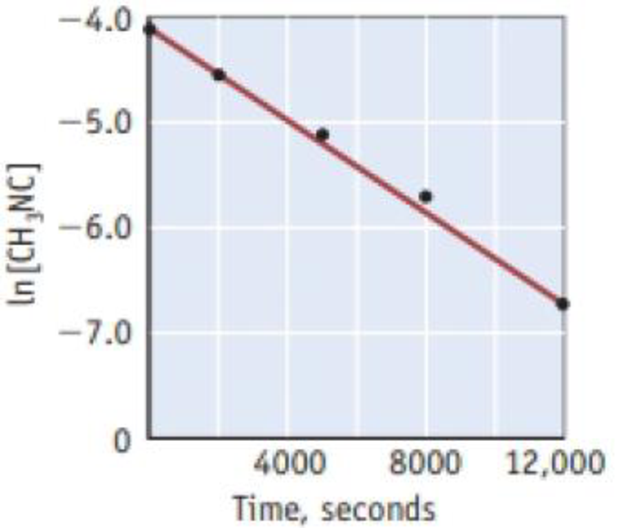# Isomerization of CH 3 NC occurs slowly when CH 3 NC is heated. CH 3 NC(g) → CH 3 CN(g) To study the rate of this reaction at 488 K, data on [CH 3 NC] were collected at various times. Analysis led to the following graph. (a) What is the rate law for this reaction? (b) What is the equation for the straight line in this graph? (c) Calculate the rate constant for this reaction. (d) How long does it take for half of the sample to isomerize? (e) What is the concentration of CH 3 NC after 1.0 × 10 4 s?### Chemistry & Chemical Reactivity

9th Edition
John C. Kotz + 3 others
Publisher: Cengage Learning
ISBN: 9781133949640

#### Solutions

Chapter
Section### Chemistry & Chemical Reactivity

9th Edition
John C. Kotz + 3 others
Publisher: Cengage Learning
ISBN: 9781133949640
Chapter 14, Problem 54GQ
Textbook Problem
304 views

## Isomerization of CH3NC occurs slowly when CH3NC is heated.CH3NC(g) → CH3CN(g)To study the rate of this reaction at 488 K, data on [CH3NC] were collected at various times. Analysis led to the following graph. (a) What is the rate law for this reaction? (b) What is the equation for the straight line in this graph? (c) Calculate the rate constant for this reaction. (d) How long does it take for half of the sample to isomerize? (e) What is the concentration of CH3NC after 1.0 × 104 s?(a)

Interpretation Introduction

Interpretation:

Rate law for the reaction has to be determined.

Concept Introduction:

Rate law or rate equation: Rate law:

It is generally the rate equation that consists of the reaction rate with the concentration or the pressures of the reactants and constant parameters.

aA + bBxXRate of reaction = k [A]m[B]n

Order of a reaction: Order of a reaction is defined as the sum of exponents of the concentrations in the rate law of the given reaction.

For a reaction,

• If the plot drawn between concentration of reactant and time is linear, then it is a zeroth order reaction
• If the plot drawn between ln[reactant] and time is linear, then it is a first order reaction
• If the plot drawn between 1/[reactant] and time is linear, then it is a second order reaction
• For the zeroth and first order reaction, the graph has a negative slope whereas the graph has a positive slope for a second order reaction.

The rate of the reaction is equal to the slope of the reaction

### Explanation of Solution

The graph is given below:

The graph plotted between ln [CH3NC] and time is linear so it is a first order reaction

(b)

Interpretation Introduction

Interpretation:

Equation for the straight line has to be determined from the graph.

Concept Introduction:

Order of a reaction: Order of a reaction is defined as the sum of exponents of the concentrations in the rate law of the given reaction.

The equation for the straight line is y=mx+c

Where,

m and c are slope and intercept respectively

(c)

Interpretation Introduction

Interpretation:

Rate constant for the reaction has to be determined.

Concept Introduction:

Rate law or rate equation: Rate law:

It is generally the rate equation that consists of the reaction rate with the concentration or the pressures of the reactants and constant parameters.

aA + bBxXRate of reaction = k [A]m[B]n

Rate constant, k: The rate constant for a chemical reaction is the proportionality term in the chemical reaction rate law which gives the relationship between the rate and the concentration of the reactant present in the chemical reaction.

The value of rate constant is 2.25×104s1

(d)

Interpretation Introduction

Interpretation:

Time required for half of the sample to isomerize has to be determined.

Concept Introduction:

Half–lifet1/2: The half–life period is defined as the time required by the reactant to reduce its concentration to one half of its initial concentration.

The half–life period for first order reaction is as follows:

t1/2=0.693kWhere,k = Rate constantt1/2= half-life

(e)

Interpretation Introduction

Interpretation:

Concentration of CH3NC after 1.0 ×104s has to be calculated.

Concept introduction:

Rate law or rate equation: Rate law:

It is generally the rate equation that consists of the reaction rate with the concentration or the pressures of the reactants and constant parameters.

aA + bBxXRate of reaction = k [A]m[B]n

Order of a reaction: Order of a reaction is defined as the sum of exponents of the concentrations in the rate law of the given reaction.

For a reaction,

• If the plot drawn between concentration of reactant and time is linear, then it is a zeroth order reaction
• If the plot drawn between ln[reactant] and time is linear, then it is a first order reaction
• If the plot drawn between 1/[reactant] and time is linear, then it is a second order reaction
• For the zeroth and first order reaction, the graph has a negative slope whereas the graph has a positive slope for a second order reaction.

### Still sussing out bartleby?

Check out a sample textbook solution.

See a sample solution

#### The Solution to Your Study Problems

Bartleby provides explanations to thousands of textbook problems written by our experts, many with advanced degrees!

Get Started

Find more solutions based on key concepts
Efforts in all of the following realms are necessary for weight change a. eating patterns, physical activity an...

Nutrition: Concepts and Controversies - Standalone book (MindTap Course List)

What is the structure and function of a mitochondrion?

Biology: The Dynamic Science (MindTap Course List)

What are the Milky Way Galaxys spiral arms?

Foundations of Astronomy (MindTap Course List)

Review. Why is the following situation impossible? An athlete tests her hand strength by having an assistant ha...

Physics for Scientists and Engineers, Technology Update (No access codes included)